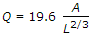# Civil Engineering - Water Resources Engineering

### Exercise :: Water Resources Engineering - Section 1

46.

Consumptive use of a crop during growth, is the amount of

 A. interception B. transpiration C. evaporation D. all the above.

Explanation:

No answer description available for this question. Let us discuss.

47.

For calculating the evaporation rate over a reservoir surface E = 0.771 (1.465 - 0.00732 B) (0.44 - 0.007375 V) (pe - pa), the equation is given by

 A. Roohwer's, formula in M.K.S. B. Roohwer's formula in F.P.S. C. Dalton's formula in F.P.S. D. Dalton's formula in M.K.S.

Explanation:

No answer description available for this question. Let us discuss.

48.

For the estimate of high floods in fan-shaped catchment, the formula used is

 A. Dicken's formula B. Ryve's formula C. Inglis formula D. none of these.

Explanation:

No answer description available for this question. Let us discuss.

49.

Burge formulacumecs is based upon

 A. rainfall and drainage area B. run off and drainage area C. drainage area and its shape D. drainage area.